4.8 Ratio and Proportions:

4.8 .1 Introduction:

We come across situations where we need to distribute income/share profit in unequal proportions.

Sometimes, we may have to compare performance of teams and individuals (For example a batsmen in a cricket match has scored more than twice the score of another player)

In 6.4, we have seen that the centroid cuts the median in unequal terms (two to one).

Under these circumstances study of the concept ‘ratio and proportion’ is useful.

The ratio of two to one is written as 2:1.

Similarly the ratio of a to b is written as a:b.

If a and b are two non zero quantities of same unit and same kind, then the fraction a/b is called the ‘ratio’ of a to b and is written as a:b (we can also express a/b=k).

a and b are called ‘terms’ of the ratio. Moreover the first term a is called ‘antecedent’ and the second term b is called ‘consequent’.

While comparing two quantities in terms of ratio, we need to note the following:

1.      Ratio is always a pure number and does not have any unit of measurement.

2.      Since ratios are pure numbers, they follow the normal rules of addition/subtraction/multiplication/division of real numbers.

3.      Ratio is normally expressed in its lowest terms (10:40 is expressed  as 1:4)

4.      When we compare two quantities, they must be converted to same unit of measurement before comparison

(For example if 1 hour 15 minutes and 45 minutes are to be compared, both of them need to be converted to hours or minutes)

Properties of ratios:

1. If a:b then for m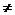0, ma:mb and a/m = b/m (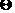they all represent the same fraction a/b)

2. Given two ratios a:b = c:d  then ad = bc (a/b = c/d)

3. Given two ratios a:b > c:d  then ad > bc (a/b > c/d)

4. Given two ratios a:b < c:d  then ad < bc (a/b < c/d)

Example: Can you solve the following problem?

The marks obtained by 4 students in an examination are as follows:

Ram:Shyam =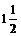: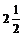Shyam:Gopal =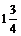: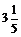Gopal:Rama =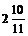: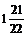Find the ratio of marks of Ram:Shyam:Gopal:Rama. If Ram had got 42 marks find the marks of others.

Working:

Since each individual ratio do not have common terms, we need to have common terms for comparison.

Ram:Shyam =:= 3/2:5/2 = 3:5 (LCM of denominators of two ratios are 2)

= 21:35 (by multiplying both terms of the ratio by 7)

Shyam:Gopal =:= 7/4:16/5 = 35/20:64/20 = 35:64 (LCM of denominators of two ratios are 20)

Gopal:Rama =:= 32/11: 43/22 = 64/22:43/22=64:43  (LCM of denominators of two ratios are 22)

Thus

Ram : Shyam : Gopal : Rama= 21:35:64:43

Since 42 is Ram’s mark and 42 = 21*2 we multiply each term of the ratio by 2

Hence

Ram : Shyam : Gopal : Rama= 21:35:64:43= 21*2:35*2:64*2:43*2

Thus their marks are 42, 70, 128 and 86 respectively.

4.8 Problem 1: Present ages of sister and brother is 14 years and 10 years respectively. After how many years will the ratio of their age becomes 5:4?

Solution:

Let x be the number of years after which the ratio becomes 5:4.

After x years sister’s age will be 14+x and brother’s age will be 10+x

It is given that the ratio after x years will be 5:414+x/10+x = 5/44(14+x) = 5(10+x) (By cross multiplication)56+4x = 50+5xx = 6 (By transposition)

Verification: After 6 years sister’s age will be 20 and brother’s age will be 16 and 20:16 = 5:4 which is as given in the problem and hence the solution is correct.

4.8 Problem 2: An ancient art piece of alloy weighing 10kg has 65% copper. Another piece of alloy weighing 15kg contains 70% copper. The two pieces are melted together and a new piece of alloy is cast. What is the % of copper in the new piece thus cast?

Solution:

First we need to find the mass of copper in both alloys.

Let copper be x kg in the first alloyx/10 = 65/100x =6.5kg

Let copper be y kg in the second alloyy/15 = 70/100y =10.5kg

Hence in the new piece of 25kg (10kg+15kg) mass of copper is 17kg (6.5kg+10.5kg)

Therefore the ratio of mass of copper to alloy is 17:25

But 17/25 =68/100

Therefore the new piece contains 68% copper.

4.8 .2 Properties of equal ratio:

If 4:5 = 8:10 we notice that the following are all true

 No Reason 1 5:4 = 10:8 5/4 =10/8 2 4:8 = 5:10 4/8=5/10 3 (4+5)/5 =(8+10)/10 9/5 =18/10 4 (4-5)5 =(8-10)/10 -1/5 = -2/10 5 (4+5)/(4-5) =(8+10)/(8-10) 9/(-1) = 18/(-2)

Did you notice that the ratios can be negative also?

In general, if a:b = c:d (a/b=c/d) then the following are all true (m is any real number)

 No Equivalent Ratio Property name Proof Which means or implies 1 b:a = d:c Invertendo b/a = d/c bc=ad 2 a:c = b:d Alternendo a/c =b/d ad=bc 3 (a+mb):b = (c+md):d Componendo (a/b)+m = (c/d)+m (a+mb)/b = (c+md)/d or (a+mb)/(c+md) = b/d 4 (a-mb):b = (c-md):d Dividendo (a/b)-m = (c/d)-m (a-mb)/b = (c-md)/d  or (a-mb)/(c-md) = b/d 5 (a+mb):(a-mb) = (c+md):(c-md) Componendo - Dividendo (a+mb)/(c+md) = b/d =(a-mb)/(c-md) From ratio in Sl No 3 and 4

4.8 Problem 3: If (x+3y)/(x+y) =3/2 then find

x/y, x2+y2/ x2-y2 , x3+y3/ x3-y3

Solution:

(x+3y)/(x+y) =3/2 (given)

By Componendo – Dividendo,

(x+3y)+(x+y)/(x+3y)-(x+y) = (3+2)/(3-2) = 5/1(2x+4y)/2y = 5/12x+4y = 10y2x = 6yx/y = 6/2 = 3/1x2/y2 = 9/1; x3/y3= 27/1

Since x2/y2 = 9/1By Componendo – Dividendo,

x2+y2/ x2-y2  = (9+1)/(9-1) = 10/8 =5/4

Since x3/y3= 27/1By Componendo – Dividendo,

X3+y3/ x3-y3 = (27+1)/(27-1) = 28/26 =14/13

4.8 Problem 4: Solve the following equations:

(x2-16x+63)/ (x2-6x+8) = (x2-16x+60)/ (x2-6x+5)

Solution:

By Alternendo

(x2-16x+63)/(x2-16x+60) = (x2-6x+8)/(x2-6x+5)

By Dividendo

{(x2-16x+63)-(x2-16x+60)}/ (x2-16x+60) = {(x2-6x+8)-(x2-6x+5)}/ (x2-6x+5)3/(x2-16x+60) = 3/(x2-6x+5)(x2-16x+60) = (x2-6x+5)-10x = -55 (By transposition)x = 11/2

4.8 Problem 5: The work done by (x-3) men in (2x+1) days and the work done by (2x+1) men in (x+4) days are in the ratio of 3:10. Find the value of x.

Solution:

A man day is defined as the unit of work done by one person in one day.

Work done by (x-3) men in (2x+1) days = [(x-3)(2x+1)] man days

Work done by (2x+1) men in (x+4) days = [(2x+1) (x+4)] man days

Assuming that the work done is same, we can say that

[(x-3)(2x+1)]/ [(2x+1) (x+4)] = 3/10

Since (2x+1) cannot be zero (if it is 0 then the number of men will be half which can not be true), we can cancel common factors in LHS of the above statement.

[(x-3)(2x+1)]/ [(2x+1)(x+4)]= 3/10

Thus

(x-3)/(x+4) =3/10

10x-30 = 3x+12

7x = 42x = 6

4.8 .3 Theorem on equal ratio:

If a/b = c/d = e/f = g/h …….. and k, l, m, n, … are any numbers then

a/b = c/d = e/f = (ak+cl+em+gn…….)/(bk+dl+fm+hn….)

Proof:

Let a/b = c/dad = bc

Let us take the term a(bk+dl)

a(bk+dl)

=abk+bcl (bc=ad)

=b(ak+cl)  (Take the common factor ‘b’ out)a(bk+dl) = b(ak+cl)a/b = (ak+cl)/(bk+dl)

By extending this proof from two terms to more  number of terms we have

a/b = c/d = e/f = (ak+cl+em+gn…….)/(bk+dl+fm+hn….)

4.8 Problem 6: Solve (12x2-20x+21)/ (4x2+4x+15) = (3x-5)/(x+1)

Solution:

We can observe that LHS has terms in x2 but RHS has terms only in x. Also we may note that if we multiply both the numerator and the denominator of RHS by 4x

we get terms in x2. Thus it is logical to multiply both numerator and denominator of RHS by 4x.

However, we can only do this  when x0

Can x be zero?

If x = 0 then LHS = 21/15 and RHS = -5/1

Since LHSRHS, x cannot be zero.

RHS = {(3x-5)/(x+1)}*(4x/4x) = (12x2-20x)/(4x2+4x)The given equation can be rewritten as follows with each ratio being equal to k.

(12x2-20x+21)/(4x2+4x+15) = (12x2-20x)/(4x2+4x) = k

By theorem on equal ratios

{(12x2-20x+21) - (12x2-20x)} / {(4x2+4x+15)- (4x2+4x)} = k21/15 = k=7/5

But k = (3x-5)/(x+1)7/5 = (3x-5)/(x+1)7x+7 = 15x-2532=8x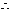x=4

Verification:   substitute x=4 in the given equation

LHS = (192-80+21)/(64+16+15) = 133/95=7/5

RHS = (12-5)/(4+1) = 7/5

Since LHS=RHS our solution is correct.

4.8 Problem 7: If y/(b+c-a) = z/(c+a-b) = x/(a+b-c) then prove that a/(z+x) = b/(x+y) = c/(y+z)

Solution:

We notice that the given ratios have x, y and z in numerator and a, b and c in denominator. But the ratios we need to prove have a, b and c in numerator and x, y and z in denominator. Thus our working will be easy if we do invertendo of the given ratios.By invertendo and making each ratio equal to k we have

(b+c-a)/y = (c+a-b)/z = (a+b-c)/x = k

By applying the theorem on equal ratios on last two terms we have

k = {(c+a-b)+(a+b-c)}/(z+x) = 2a/(x+z)

Similarly we can prove k = 2b/(x+y) and k = 2c/(y+z)k = 2a/(x+z) =2b/(x+y) = 2c/(y+z)

By dividing each of the above ratios by 2 we get

a/(x+z) = b/(x+y) = c/(y+z)

4.8 .3 Proportion:

If a/b = c/d then the numbers a, b, c and d are said to be in proportion and we write them as a:b::c:d. In such a relationship we call a and d as ‘extremes’ and b and c as middle terms or ‘means’

What if a/b = b/c (i.e. a:b = b:c) such that

b2 = ac and b = SQRT(ac)

In such  case we say a, b and c are in ‘continued proportion’ and b is called geometric mean or mean proportional between a and c

4.8 Problem 8:  The middle of three numbers in continued proportion is 24 and the sum of first and the third is 52. Find the numbers.

Hint:

Let a and c be the first and the third number. Thus the three numbers in continued proportion are a, 24 and c.ac = 242=576

It is also given that a+c = 52c =52-a

By substituting this value in ac we get the following equation to solve

a2-52a+576 = 0

After factorisation we find that a=16 and a=36 are the roots of the equation and consequently 36 or 16 are the values of c respectively.

Hence (16,24,36) or (36,24,16) are the three required numbers.

4.8 .4  k-method:

In this method we equate each of the ratio to a number k and then solve the problem. Hence this method is called as k-method.

4.8 Problem 9:  Five numbers are in continued proportion. The product of the 1st and the 5th of them is 324 and the sum of the 2nd and the 4th is 60. Find the numbers.

Hint:

Let a, b, c, d and e be the numbers.

It is given that ae=324 and b+d=60

Let the ratio of the numbers be k

If we can find the value of k we could also find the value of the numbers

a/b=b/c=c/d=d/e=k

d=ek, c=dk=ek2, b=ck=ek3 and a=bk=ek4

Let us take a=ek4(324/e) = ek4 (ae = 324)324 = e2k4 (324=18*18)e =18/k2

ek3+ek = 60 (b+d=60)(18/k2)k3+(18/k2)k=60 (e=18/k2)18k + 18/k = 603k+3/k=103k2-10k+3=0k = 3 and  k = 1/3 are the roots of the above equation

If k = 3 then we have e=2, a=162, b=54, c=18 and d=6

If k =1/3 then we have e=162, a=2, b=6, c=18 and d=54The required numbers are (162,54,18,6,2) or (2,6,18,54,162).

4.8 Problem 10:  If a:b::c:d show that

1. (a+b):(c+d) = SQRT(a2+b2): SQRT(c2+d2)

2. (a2+c2):(ab+cd) = (ab+cd):(b2+d2)

Solution:

Let a/b = c/d = ka = bk, c = dk

Part 1:

LHS = (bk+b)/(dk+d) = b/d

RHS = SQRT(b2k2+b2)/SQRT(d2k2+d2)

= b.SQRT(k2+1)/ d.SQRT(k2+1)= b/d

Thus LHS=RHS

Part 2:

LHS = (b2k2 + d2k2)/(b2k + d2k) = k2/k = k

RHS = (b2k + d2k)/(b2  + d2) = k

Thus LHS=RHS

4.8 Summary of learning

 If a/b = c/d = e/f = g/h . . . . and k, l, m, n… are any numbers then No Points to remember 1 b:a = d:c 2 a:c = b:d 3 (a+mb):b = (c+md):d 4 (a-mb):b = (c-md):d 5 (a+mb):(a-mb) = (c+md):(c-md) 6 a:b = c:d = e:f = (ak+cl+em+gn..):(bk+dl+fm+hn. .)